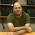## Sunday, December 31, 2017

### Statistics Sunday: Different Means

While reading a history of mathematics book earlier this year, I was surprised to learn about the number of means one can compute to summarize values. I was familiar with the common descriptive statistics for central tendency - mean, median, and mode - and was aware that this particular mean is also called the arithmetic mean, so I suspected there were more kinds of means. But I wasn't exposed to any of them beyond arithmetic mean in my statistics classes.

I've started to do some research into the different kinds of means to share here. Today, I'll start with the geometric mean.

The arithmetic mean, of course, is calculated by adding together all values then dividing by the number of values. But there are many cases where the arithmetic mean isn't really an appropriate measure. If you're dealing with values that are serially correlated - there is shared variance between values of a variable over time - the arithmetic mean may not be the best descriptive statistic.

For instance, say you're tracking return on an investment over time. Those values will be correlated across time and you'll have compounding that must be taken into account. The geometric mean is well-suited for this situation - in fact, it's frequently used among investment professionals.

The geometric mean is calculated by multiplying n values together, then taking the nth root. As you can imagine, for a few values, this could easily be calculated by hand. For instance, to demonstrate, let's say I have 5 values - return over the last 5 years:

Year 1 - 1%
Year 2 - 7%
Year 3 - -2%
Year 4 - 6%
Year 5 - 3%

For a \$100 investment, the value over the 5 years would be:

Year 1 - \$100 * 1.01 = \$101.00
Year 2 - \$101 * 1.07 = \$108.07
Year 3 - \$108.07 * 0.98 = \$105.91
Year 4 - \$105.91 * 1.06 = \$112.26
Year 5 - \$112.26 * 1.03 = \$115.63

The arithmetc mean of these 5 return rates (1.01, 1.07, 0.98, 1.06, and 1.03) would be 1.03, or a 3.0% rate of return. The geometric mean would be the product of these 5 values (approximately 1.156) taken the 5th root = 1.029 or 2.9%. Pretty close. If we had more values and/or more volatility in those values, we might see more of a discrepancy between the values. One thing to note, though, is that the geometric mean will be less than or equal to the arithmetic mean; it won't be greater than it.

For large ns, you'll want to have a computer do this calculation for you. (The same could be said for the arithmetic mean, I suppose.)

Fortunately, many data analysis programs offer a geometric mean calculation. You can compute this in Excel for up to 255 values using the GEOMEAN function. SPSS offers geometric mean in the Analyze->Compare Means->Means option. And the psych package for R offers a geometric.mean function.

Today, I'm looking back over my own data for the year - books read, blog posts written, writing accomplished, and so on - and generating some metrics to describe 2017 for me. I may not need to take the geometric mean of anything, but it's always good to have different descriptive statistics in your back pocket. You never know when they might be useful. Look for a "measurement" post sometime today or tomorrow.

Happy new year, everyone! Have a fun celebration tonight, stay safe, and I'll see you in 2018!

A few edits: 1) As Jay points out below, geometric mean isn't so great if a value is 0 or negative. Any value times 0 is of course 0, and any positive value times a negative value is negative. So your result will be meaningless if you have 0s or negatives.
2) My friend, David, over at The Daily Parker let me know that this isn't how he learned to compute rate of return in business school. This is probably a good demonstration that there are many ways to summarize a set of values, and also a demonstration that I don't really know a lot about investment or economics. I love numbers, but not so much numbers with \$ signs in front of them. Mostly I wanted to share how to compute geometric mean and I based my example of a few different examples I saw on the internet. (Yes, I know, just because it's on the internet doesn't mean it's correct.) So if the investment example is incorrect or meaningless, I'm okay with that. But the geometric mean can be well-suited for other applications, as long as you watch out for #1 above.
Thank you both for your feedback!

#### 1 comment:

1.A few things to note:

-The geometric mean is only meaningful for ratio-scale data. A value of 0 is an edge case where the GM will equal 0 regardless of all other values, but this is unlikely to be a desirable feature of it.
-To compute the GM, you can take the natural logs of the data, compute the arithmetic mean of those, and then exponentiate. No need for a custom function.